# The rating of the best interest rates under the term deposits for 2 years23.04.2021

## Bankchart.com.au has surveyed the terms of term deposits for 2 years in the national currency. The survey covered all the deposits with any form of interests repayment, regardless of the replenishment option or the deposit partial withdrawal option. Our website's analysts have studied the loan offers from all Australian banks. Review date - 23.04.2021

As of 31 March 2021 the deposits under review have been offered by 28 banks.   The average interest rate under such deposits equals 0,36 % p.a. The total number of the deposits programs available for the specified terms is 109.

The maximal return among the deposits under review is offered by BankVic. The interest rate under "Term deposit" deposit program constitutes 0,90 % p.a. The table below shows the best offers by each bank selected by Bankchart.com.au (in terms of profitability) for the aforementioned terms.

### The TOP-28 term deposits for 2 years, as of 31 March 2021

#

Name

Interest Rate, %

1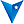BankVic

«Term deposit»

0,90

interest rate, %

rate type

fixed

interest periodicity

at maturity

minimum sum

\$ 5 000,00

2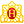Heritage Bank

«Term Deposits»

0,80

interest rate, %

rate type

fixed

interest periodicity

annually

minimum sum

\$ 1 000,00

3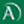Arab Bank

«Fixed Term Deposit»

0,80

interest rate, %

rate type

fixed

interest periodicity

at maturity

minimum sum

\$ 50 000,00

4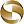Mega International Commercial Bank

«Term Deposits»

0,70

interest rate, %

rate type

fixed

interest periodicity

annually

minimum sum

\$ 1 000,00

5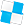Police Bank

«Term Deposits»

0,65

interest rate, %

rate type

fixed

interest periodicity

every 6 months

minimum sum

\$ 10 000,00

6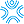Bank First

«Term Deposits»

0,65

interest rate, %

rate type

fixed

interest periodicity

monthly

minimum sum

\$ 50 000,00

7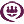RACQ

«Term Deposit»

0,65

interest rate, %

rate type

fixed

interest periodicity

monthly

minimum sum

\$ 1 000,00

8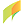G&C Mutual Bank

«Term Deposits»

0,60

interest rate, %

rate type

fixed

interest periodicity

at maturity, annually

minimum sum

\$ 1 000,00

9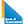Bank of Queensland

«Term deposit»

0,60

interest rate, %

rate type

fixed

interest periodicity

at maturity

minimum sum

\$ 5 000,00

10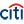Citibank

«Term deposit»

0,50

interest rate, %

rate type

fixed

interest periodicity

monthly

minimum sum

\$ 250 000,00

11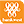Bankwest

«Online Term Deposit»

0,50

interest rate, %

rate type

fixed

interest periodicity

at maturity, annually

minimum sum

\$ 5 000,00

12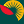Suncorp Bank

«Term Deposit»

0,50

interest rate, %

rate type

fixed

interest periodicity

at maturity

minimum sum

\$ 1 000 000,00

13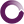Beyond Bank Australia

«Term Deposits»

0,50

interest rate, %

rate type

fixed

interest periodicity

annually

minimum sum

\$ 200 000,00

14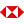HSBC Bank

«Term Deposits»

0,50

interest rate, %

rate type

fixed

interest periodicity

at maturity, annually

minimum sum

\$ 5 000,00

15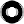Macquarie Bank

«Term Deposit»

0,45

interest rate, %

rate type

fixed

interest periodicity

monthly

minimum sum

\$ 0,00

16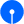State Bank of India

«Term Deposits»

0,40

interest rate, %

rate type

fixed

interest periodicity

at maturity

minimum sum

\$ 100,00

17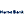Hume Bank

«Term Deposits»

0,40

interest rate, %

rate type

fixed

interest periodicity

at maturity, annually

minimum sum

\$ 5 000,00

18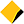Commonwealth Bank of Australia

«Term Deposits»

0,35

interest rate, %

rate type

fixed

interest periodicity

annually

minimum sum

\$ 50 000,00

19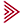Bendigo and Adelaide Bank

«Term deposit»

0,30

interest rate, %

rate type

fixed

interest periodicity

at maturity

minimum sum

\$ 5 000,00

20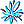AMP Bank

«Term Deposits»

0,30

interest rate, %

rate type

fixed

interest periodicity

at maturity

minimum sum

\$ 500 000,00

21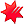National Australia Bank

«NAB Term Deposits»

0,30

interest rate, %

rate type

fixed

interest periodicity

at maturity, annually

minimum sum

\$ 5 000,00

22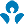Australia and New Zealand (ANZ)

0,30

interest rate, %

rate type

fixed

interest periodicity

quarterly

minimum sum

\$ 5 000,00

23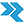Rural Bank

«Long Term Deposit»

0,25

interest rate, %

rate type

fixed

interest periodicity

annually

minimum sum

\$ 5 000,00

24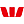Westpac Bank

«Term Deposit»

0,25

interest rate, %

rate type

fixed

interest periodicity

monthly

minimum sum

\$ 5 000,00

25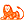ING Bank

«Personal Term Deposit»

0,25

interest rate, %

rate type

fixed

interest periodicity

at maturity

minimum sum

\$ 10 000,00

26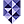Bank of Melbourne

«Term Deposits»

0,25

interest rate, %

rate type

fixed

interest periodicity

monthly

minimum sum

\$ 1 000,00

27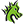St. George Bank

«Term Deposits»

0,25

interest rate, %

rate type

fixed

interest periodicity

at maturity, annually

minimum sum

\$ 1 000,00

28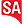Bank SA

«Term Deposits»

0,25

interest rate, %

rate type

fixed

interest periodicity

at maturity

minimum sum

\$ 1 000,00

To view the up-to-date rating of the deposits at our website here.

Do you want to know by email when we update the rates?